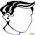## 2011/12/22

### Code

```@Override public void mouseClicked(MouseEvent e) { JTableHeader header = (JTableHeader)e.getSource(); JTable table = header.getTable(); TableColumnModel columnModel = table.getColumnModel(); int vci = columnModel.getColumnIndexAtX(e.getX()); int mci = table.convertColumnIndexToModel(vci); TableColumn column = table.getColumnModel().getColumn(mci); Rectangle r = header.getHeaderRect(vci); Container c = (Container)getTableCellRendererComponent(table, "", true, true, -1, vci); r.translate(r.width-BUTTON_WIDTH, 0); r.setSize(BUTTON_WIDTH, r.height); Point pt = e.getPoint(); if(c.getComponentCount() > 0 && r.contains(pt) && pop!=null) { pop.show(header, r.x, r.height); JButton b = (JButton)c.getComponent(0); b.doClick(); e.consume(); } } @Override public void mouseExited(MouseEvent e) { rolloverIndex = -1; } @Override public void mouseMoved(MouseEvent e) { JTableHeader header = (JTableHeader)e.getSource(); JTable table = header.getTable(); TableColumnModel columnModel = table.getColumnModel(); int vci = columnModel.getColumnIndexAtX(e.getX()); int mci = table.convertColumnIndexToModel(vci); rolloverIndex = mci; } ```

### References

1.hi I like your such a paging input in java wanted to ask you how I extract the values ​​of the selected row when the table contains the paging? please help me how to do it not, is that you are a pro in java I notice in your code has a phenomenal structure is so I hope your answer thanks

2.Hi HANNIBAL
I think JTable#convertRowIndexToModel(int) is what you are looking for.

http://docs.oracle.com/javase/6/docs/api/javax/swing/JTable.html
Similarly when using the sorting and filtering functionality provided by RowSorter the underlying TableModel does not need to know how to do sorting, rather RowSorter will handle it. Coordinate conversions will be necessary when using the row based methods of JTable with the underlying TableModel. All of JTables row based methods are in terms of the RowSorter, which is not necessarily the same as that of the underlying TableModel. For example, the selection is always in terms of JTable so that when using RowSorter you will need to convert using convertRowIndexToView or convertRowIndexToModel. The following shows how to convert coordinates from JTable to that of the underlying model:
int[] selection = table.getSelectedRows();
for (int i = 0; i < selection.length; i++) {
selection[i] = table.convertRowIndexToModel(selection[i]);
}
// selection is now in terms of the underlying TableModel